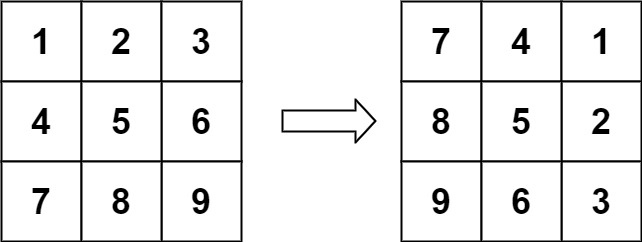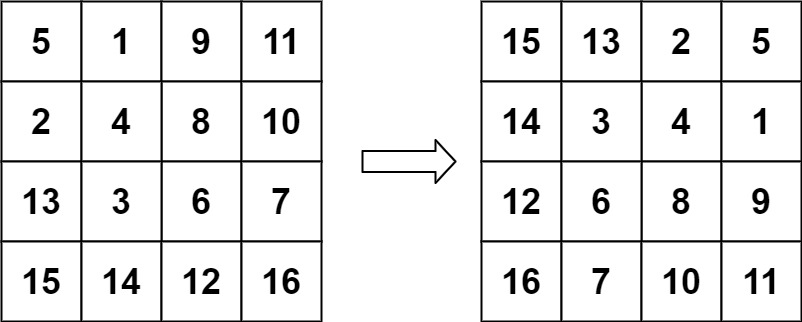# 48. Rotate Image / Medium

You are given an n x n 2D matrix representing an image, rotate the image by 90 degrees (clockwise).

You have to rotate the image in-place, which means you have to modify the input 2D matrix directly. DO NOT allocate another 2D matrix and do the rotation.

## Example 1:Input: matrix = [[1,2,3],[4,5,6],[7,8,9]]
Output: [[7,4,1],[8,5,2],[9,6,3]]

## Example 2:Input: matrix = [[5,1,9,11],[2,4,8,10],[13,3,6,7],[15,14,12,16]]
Output: [[15,13,2,5],[14,3,4,1],[12,6,8,9],[16,7,10,11]]

## Example 3:

Input: matrix = []
Output: []

## Example 4:

Input: matrix = [[1,2],[3,4]]
Output: [[3,1],[4,2]]

## Constraints:

• matrix.length == n
• matrix[i].length == n
• 1 <= n <= 20
• -1000 <= matrix[i][j] <= 1000

# Solution 1: Brute Force

## 效能

### Complexity

• Time Complexity: O(N^2)
• Space Complexity: O(1)

### LeetCode Result

• Runtime: 0 ms
• Memory Usage: 7.1 MB

# Solution 2: Transpose & Reverse

## 效能

### Complexity

• Time Complexity: O(N^2)
• Space Complexity: O(1)

### LeetCode Result

• Runtime: 0 ms
• Memory Usage: 7.1 MB